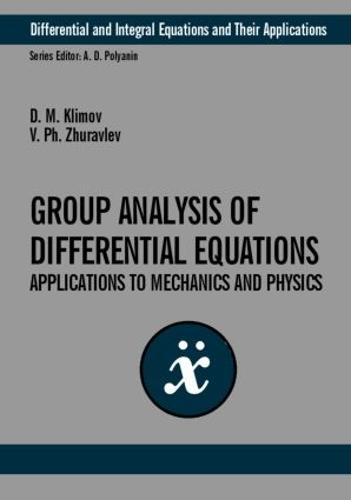•# Group-Theoretic Methods in Mechanics and Applied Mathematics - Differential and Integral Equations and Their Applications (Hardback)

(author), (author)
£100.00
Hardback 240 Pages / Published: 15/08/2002
• We can order this

Usually dispatched within 3 weeks

Group analysis of differential equations has applications to various problems in nonlinear mechanics and physics. For the first time, this book gives the systematic group analysis of main postulates of classical and relativistic mechanics. The consistent presentation of Lie group theory is illustrated by plentiful examples. Symmetries and conservation laws of differential equations are studied. Specific equations and problems of mechanics and physics are considered, and exact solutions are given for the following equations: dynamics of rigid body, heat transfer, wave, hydrodynamics, Thomas-Fermi and more. The author pays particular attention to the application of group analysis to developing asymptotic methods of applied mathematics in problems with small parameter. The methods are used to solve basic equations (Van Der Pol's equation, Duffing equation, etc.) encountered in the theory of nonlinear oscillations. This book is intended for a wide range of scientists, engineers and students in the fields of applied mathematics, mechanics and physics.

Publisher: Taylor & Francis Ltd
ISBN: 9780415298636
Number of pages: 240
Weight: 263 g
Dimensions: 254 x 178 x 18 mm